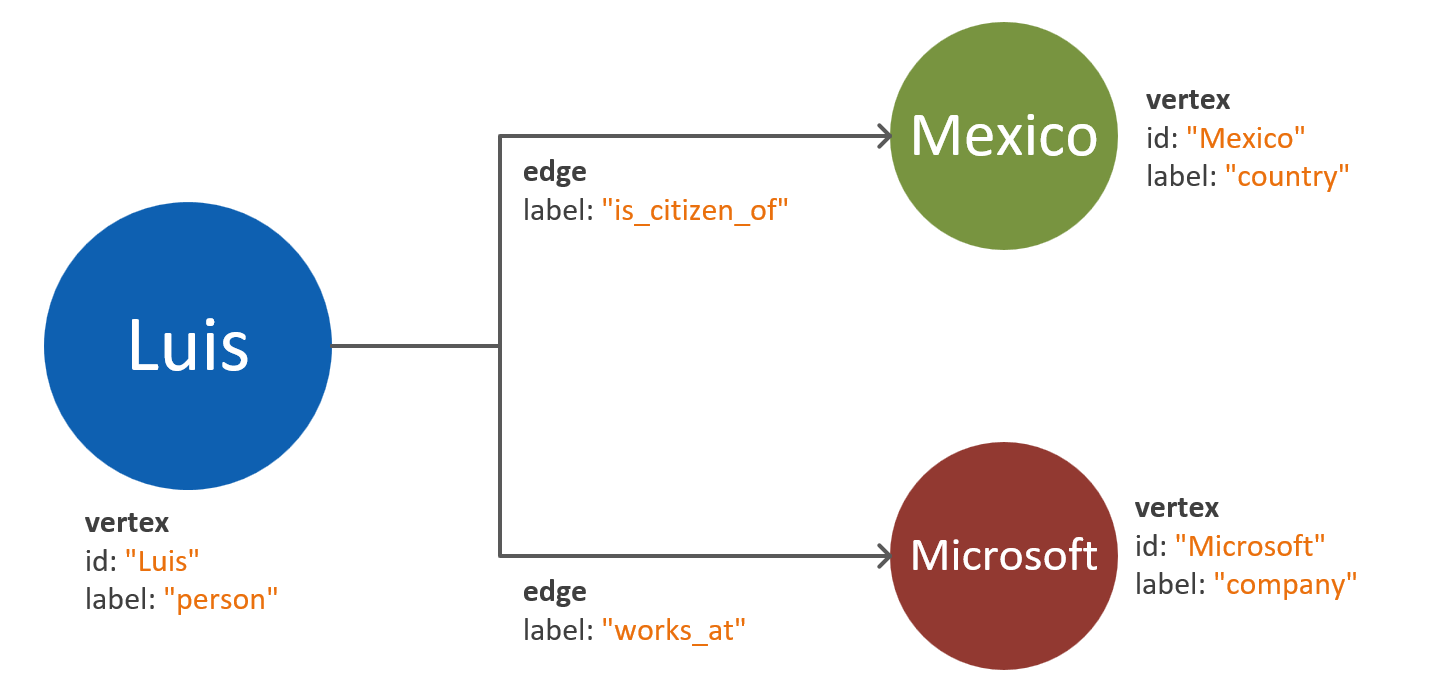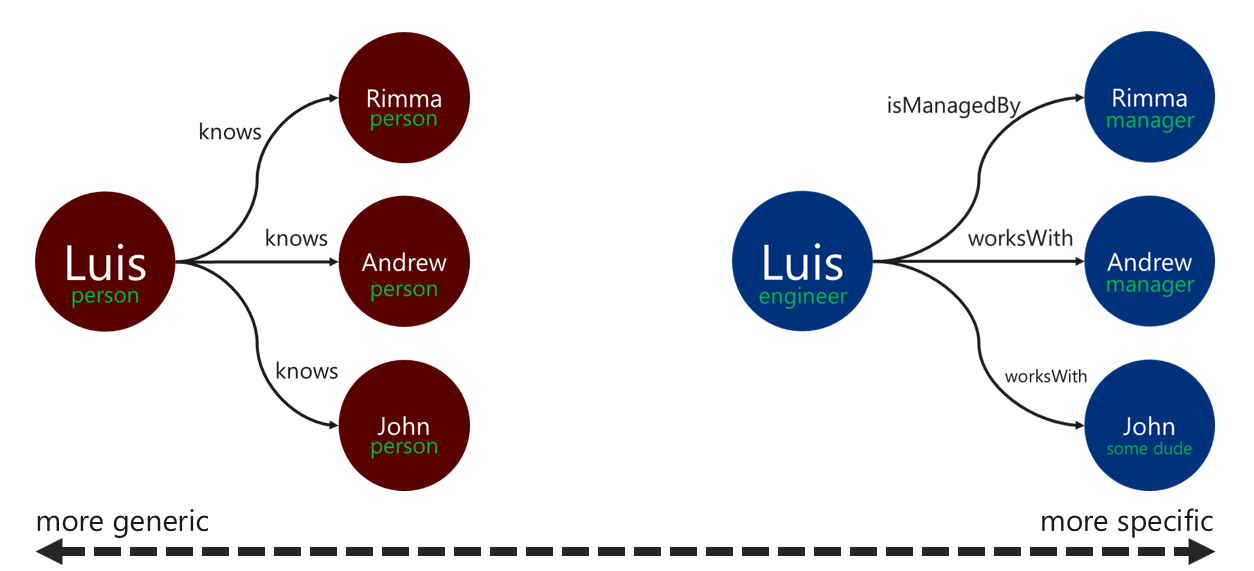# Graph data modeling with Azure Cosmos DB for Apache Gremlin

APPLIES TO:Gremlin

This article provides recommendations for the use of graph data models. These best practices are vital for ensuring the scalability and performance of a graph database system as the data evolves. An efficient data model is especially important for large-scale graphs.

## Requirements

The process outlined in this guide is based on the following assumptions:

• The entities in the problem-space are identified. These entities are meant to be consumed atomically for each request. In other words, the database system isn't designed to retrieve a single entity's data in multiple query requests.
• There's an understanding of read and write requirements for the database system. These requirements guide the optimizations needed for the graph data model.
• The principles of the Apache Tinkerpop property graph standard are well understood.

## When do I need a graph database?

A graph database solution can be optimally used if the entities and relationships in a data domain have any of the following characteristics:

• The entities are highly connected through descriptive relationships. The benefit in this scenario is that the relationships persist in storage.
• There are cyclic relationships or self-referenced entities. This pattern is often a challenge when you use relational or document databases.
• There are dynamically evolving relationships between entities. This pattern is especially applicable to hierarchical or tree-structured data with many levels.
• There are many-to-many relationships between entities.
• There are write and read requirements on both entities and relationships.

If the above criteria are satisfied, a graph database approach likely provides advantages for query complexity, data model scalability, and query performance.

The next step is to determine if the graph is going to be used for analytic or transactional purposes. If the graph is intended to be used for heavy computation and data processing workloads, it's worth exploring the Cosmos DB Spark connector and the GraphX library.

## How to use graph objects

The Apache Tinkerpop property graph standard defines two types of objects: vertices and edges.

The following are best practices for the properties in the graph objects:

Object Property Type Notes
Vertex ID String Uniquely enforced per partition. If a value isn't supplied upon insertion, an auto-generated GUID is stored.
Vertex Label String This property is used to define the type of entity that the vertex represents. If a value isn't supplied, a default value vertex is used.
Vertex Properties String, boolean, numeric A list of separate properties stored as key-value pairs in each vertex.
Vertex Partition key String, boolean, numeric This property defines where the vertex and its outgoing edges are stored. Read more about graph partitioning.
Edge ID String Uniquely enforced per partition. Auto-generated by default. Edges usually don't need to be uniquely retrieved by an ID.
Edge Label String This property is used to define the type of relationship that two vertices have.
Edge Properties String, boolean, numeric A list of separate properties stored as key-value pairs in each edge.

Note

Edges don't require a partition key value, since the value is automatically assigned based on their source vertex. Learn more in the Using a partitioned graph in Azure Cosmos DB.

## Entity and relationship modeling guidelines

The following guidelines help you approach data modeling for an Azure Cosmos DB for Apache Gremlin graph database. These guidelines assume that there's an existing definition of a data domain and queries for it.

Note

The following steps are presented as recommendations. You should evaluate and test the final model before considering it as production-ready. Additionally, the recommendations are specific to Azure Cosmos DB's Gremlin API implementation.

### Modeling vertices and properties

The first step for a graph data model is to map every identified entity to a vertex object. A one-to-one mapping of all entities to vertices should be an initial step and subject to change.

One common pitfall is to map properties of a single entity as separate vertices. Consider the following example, where the same entity is represented in two different ways:

• Vertex-based properties: In this approach, the entity uses three separate vertices and two edges to describe its properties. While this approach might reduce redundancy, it increases model complexity. An increase in model complexity can result in added latency, query complexity, and computation cost. This model can also present challenges in partitioning.• Property-embedded vertices: This approach takes advantage of the key-value pair list to represent all the properties of the entity inside a vertex. This approach reduces model complexity, which leads to simpler queries and more cost-efficient traversals.Note

The preceding diagrams show a simplified graph model that only compares the two ways of dividing entity properties.

The property-embedded vertices pattern generally provides a more performant and scalable approach. The default approach to a new graph data model should gravitate toward this pattern.

However, there are scenarios where referencing a property might provide advantages. For example, if the referenced property is updated frequently. Use a separate vertex to represent a property that's constantly changing to minimize the amount of write operations that the update requires.

### Relationship models with edge directions

After the vertices are modeled, the edges can be added to denote the relationships between them. The first aspect that needs to be evaluated is the direction of the relationship.

Edge objects have a default direction that's followed by a traversal when using the `out()` or `outE()` functions. Using this natural direction results in an efficient operation, since all vertices are stored with their outgoing edges.

However, traversing in the opposite direction of an edge, using the `in()` function, always results in a cross-partition query. Learn more about graph partitioning. If there's a need to constantly traverse using the `in()` function, it's recommended to add edges in both directions.

You can determine the edge direction by using the `.to()` or `.from()` predicates with the `.addE()` Gremlin step. Or by using the bulk executor library for Gremlin API.

Note

Edge objects have a direction by default.

### Relationship labels

Using descriptive relationship labels can improve the efficiency of edge resolution operations. You can apply this pattern in the following ways:

• Use non-generic terms to label a relationship.
• Associate the label of the source vertex to the label of the target vertex with the relationship name.The more specific the label that the traverser uses to filter the edges, the better. This decision can have a significant effect on query cost as well. You can evaluate the query cost at any time by using the executionProfile step.